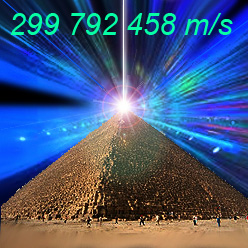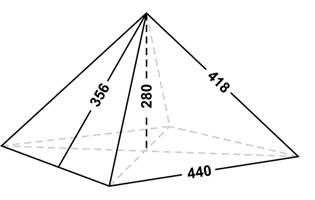# October 23, 2012## The Great Pyramid and the Speed of Light

The Great Pyramid encodes enormous amount of numerical coincidences ( pi, Phi, dimensions and movement of our planet, axial tilt, precession,  speed of light, and more…)
We can only wonder if the ancient architects were fully aware of these special numbers encoded in their design — or are these numbers simply the  result of  selecting 2 numbers (7 and 11) for proportions for the Great Pyramid???

 The design of the Great Pyramid is based on the ratio 11:7.  This ratio (equal 1.571) is perfect approximation of the “squaring the circle” principle.For the Great Pyramid, Base to Height Ratio 440/280 is exactly 11/7 Most “pyramidologists” appear to be  “chasing their tails” uncovering huge amount of “numerical coincidences” embedded in the Great Pyramid… It is simply unbelievable, however ALL of these numerical coincidences are result of selecting just 4 numbers for the pyramid design: 7, 11 (height to base ratio), 40 (the scale factor), and the 4th key number is the value of the measuring unit: Royal Cubit = 20.62 ” = 0.524 m.

This single, fundamental design principle: 11 : 7 Base to Height Ratio  generates ALL amazing mathematical properties of the Great Pyramid:

• the Golden Ratio Phi=1.618 (the Great Pyramid is a Golden Pyramid: length of the slope side (356) divided by half of the side (440/2 = 220) height is equal to 1.6181818… which is the Golden Ratio Phi
• squaring the circle ratio 1.571 (base/height = 44/28 = 1.571)
• pi=3.14159… (2 x base/height = 2 x 44/28 = 3.14286 which is very close approximation of “pi” = 3.14159…)
• Perimeter of the square base, 4×440=1760, is the same as circumference of the circle with radius = height: 2x ”pi” x height (2x 22/7 x 280=1760)
• The ratio of the perimeter to height of 1760/280 cubits equates to 2x pi
to an accuracy of better than 0.05%
• Side of the base (440) plus double height (2x 280=560) = 1,000
• Perimeter of the square base is equal 4×440=1760 RC = 0.5 nautical mile = 1/7,200th of the radius length of the earth
• the slop angle 51°.843
• The Pyramid exhibits in the design both pi and by Phi,  given the similarity
of 2/ sqrt(phi) (2 divided by the square root of Phi) with pi/2 :
• 11/ 7 equal 1.5714
• 2/ sqrt(89/55) equal 1.5722
• 2/ sqrt(Phi) equal 1.5723
• pi/ 2 equal 1.5708
• Royal Cubit = 0.5236 m,  pi – Phi2 = 0.5231
• and more…

### Does Great Pyramid encode “fractal” value of the speed of light?

The speed of light in a vacuum is 299, 792, 458 meters per second or 983,571,056.43045 feet per second or 186,282.397 miles per second.

Base of the Great Pyramid is a square with side B = 44o Royal Egyptian Cubits.  Let’s draw two circles: one inscribed and one superscribed on the square of the base of the Great Pyramid.• Circumference of superscribed circle: 2x pi x R
• Circumference of the inscribed circle: 2x pi x r

The difference of the circumference of both circles (lets call it C) is:

C = 2 x pi x (R – r) = 2 x pi x [ B/sqrt(2) – B/2 ] =
2x pi x B x [ 1/sqrt(2) – 1/2 ] = 1.301290285 x B

### The length of the Egyptian Royal Cubit

Based on The Pyramids and Temples of Gizeh” by W.M. Flinders Petrie. 1883.

The unit of measuring length used by the ancient architects in the construction of the Great Pyramid was the Royal Cubit.

Petrie estimated the value of the Royal Cubit using some key dimensions of the Great Pyramid:
By the base length of the Pyramid, if 440 cubits (section ’43): 20.611 ± .002
By the base of King’s Chamber, corrected for opening of joints:  20.632 ± .004 inches
By the Queen’s Chamber, if dimensions squared are in square cubits:  20.61   ± .020
By the antechamber: 20.58   ± .020
By the ascending and Queen’s Chamber passage lengths (section 149):  20.622 ± .002
By the gallery width:   20.605 ± .032

The Average value of the RC  (based on above numbers) is 20.61 inches.

It’s almost universally accepted that archaeologist Flinders Petrie’s determination of the royal cubit length at 20.632 inches, from his measurements of the King’s Chamber in the Great Pyramid of Giza, was the likely measure to survey the dimensions of that pyramid, 440 royal cubits per base side, but the experts stop there, not then letting you know that those 1,760 royal cubits which total the Great Pyramid’s base perimeter length, when multiplied by 20.632 inches, equals half a modern nautical mile, or 1/7,200th of the radius length of the earth, so there certainly is a connection.

By most accurate series of measurements Petrie concluded that the royal cubit standard for the Great Pyramid was 20.620 ± .005 inches ( 523.7 mm).

Let’s use the lower value 20.615 ” for the Royal Cubit  (allowed by the 0.005 ” accuracy):

the Royal Egyptian Cubit (RC) is 20.615 ” = 0.523621 m

B = 440RC = 9070.6″ = 755.883 feet = 230.393 m

Therefore C = 1.301290285 x B = 983.6 feet = 299.8 m

The value of C is surprisingly very close to
the  speed of light x 106

The speed of light in a vacuum is
983, 571, 056.43 feet per second =
299, 792, 458 meters per second.

Another very strange Great Pyramid  “coincidence” related to the speed of light was discovered by John Charles Webb Jr. :
Precise latitude of the centre of the Grand Gallery (inside GP) is
29° 58′ 45.28″ N = 29.9792458° N
The speed of light in vacuum, usually denoted by c, is a universal physical constant important in many areas of physics ( 299,792,458 metres /s ).

– – –

Another interesting coincidence encoded in the Royal Cubit:

• Royal Egyptian Cubit = 0.524 m
• pi = 3.1416
• 3.1416 feet = 0.957 m = 1.8263 RC
• 2x (0.957 / 0.524 ) = 2x  1.8263 = 3.6526 = 365.26 x 10-2

Number of days in a year: 365.25

Another interpretation of the above:
circumference of a a circle with diameter equal 100 feet is
2 x pi x 100 feet = 2 x pi x 1200″ =7539.8″ = 365.74 RC
(or 356.25 RC measured in King’s Chamber)

– – –

### The Missing Capstone Theory

The pyramidion (capstone) found near the Red Pyramid is nearly “96 cm from the top, measured along the edge“. This (and other capstones found in Egypt) suggests that Egyptians were not making huge capstones for their pyramids. Therefore assumption that missing capstone of the Great Pyramid was huge (30 feet at the base) is wrong. It is very likely that in addition to the missing pyramidion there is a layer of stones missing as well. Therefore the capstone was much smaller than the missing part of the pyramid.

To maintain perfect slope angle such a capstone would have to have identical proportions as the whole pyramid: 7:11. If we use the “Red Pyramid” capstone to estimate possible size of the capstone from the Great Pyramid, it seems reasonable to select 2 Royal Cubits (140th of the pyramid’s height)  for its height.  The capstone’s base in this case would be 3.14286 RC  (as the result of keeping the same scale and proportions as the GP) . This is very close approximation of “pi”.

The missing capstone of the Great Pyramid was very likely 2 Royal Cubits in height (3.4267 feet = 1.0475 m ) with square base of  3.1428 Royal Cubits (5.4 feet = 1.646 m).